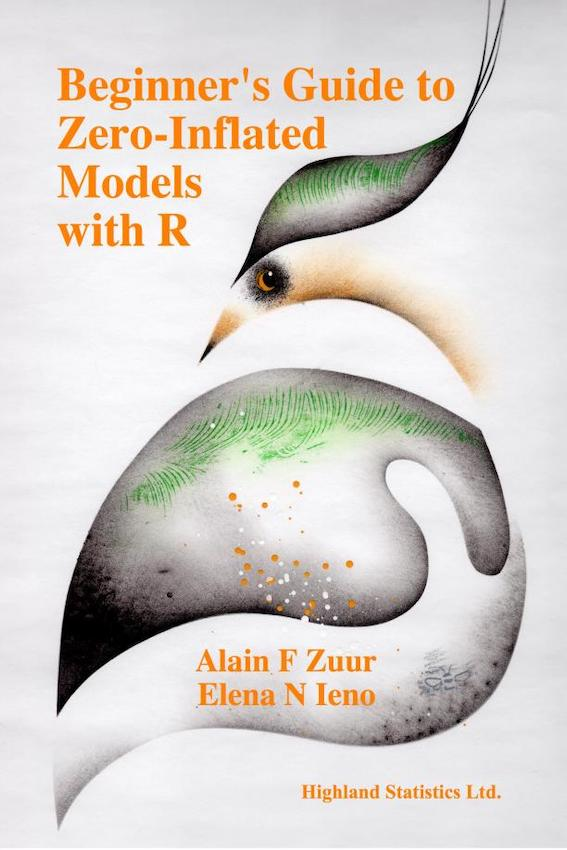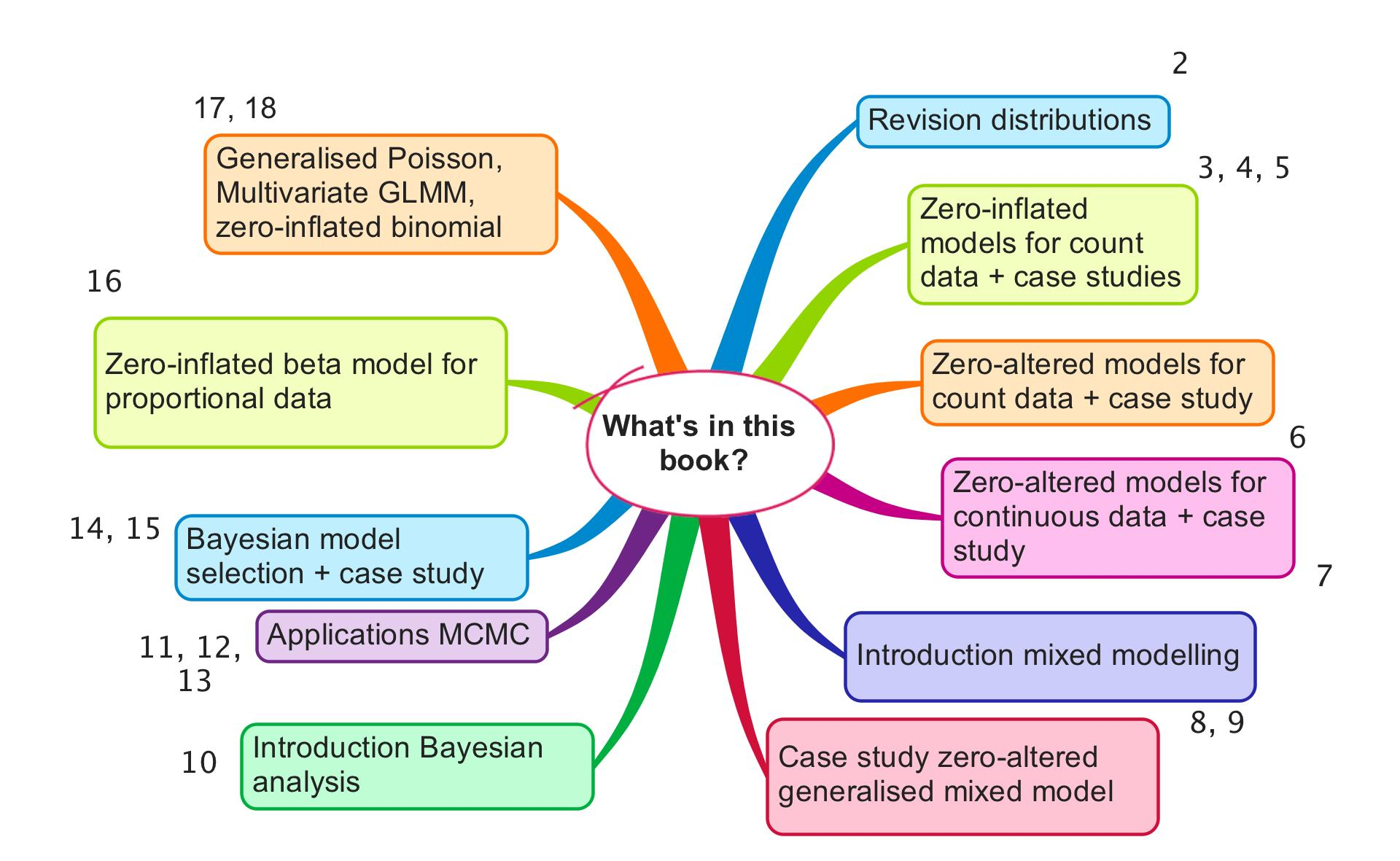#### Zuur AF and Ieno EN

In 2012 we published Zero Inflated Models and Generalized Linear Mixed Models with R. Our original plan in 2015 was to write a second edition of the 2012 book. After writing one page, we immediately decided that we had to write a completely new book.Not that we were unhappy with our 2012 book: on the contrary. But this new book, which we called Beginner’s Guide to Zero-Inflated Models with R, is, as the title suggests, intended for the beginner. This book contains only half a page of text that overlaps with the 2012 book. Everything else is new.

The minimum prerequisite for Beginner's Guide to Zero-Inflated Models with R is knowledge of multiple linear regression. In Chapter 2 we start with brief explanations of the Poisson, negative binomial, Bernoulli, binomial and gamma distributions. The motivation for doing this is that zero-inflated models consist of two distributions ‘glued’ together, one of which is the Bernoulli distribution.

We begin Chapter 3 with a brief revision of the Poisson generalised linear model (GLM) and the Bernoulli GLM, followed by a gentle introduction to zero-inflated Poisson (ZIP) models. Chapters 4 and 5 contain detailed case studies using count data of orange-crowned warblers and sharks. Just like all other chapters, these case studies are based on real datasets used in scientific papers. In Chapter 6 we use zero-altered Poisson (ZAP) models to deal with the excessive number of zeros in count data. In Chapter 7 we analyse continuous data with a large number of zeros. Biomass of Chinese tallow trees is analysed with zero-altered gamma (ZAG) models.

In Chapter 8, which begins the second part of the book, we explain how to deal with dependency. Mixed models are introduced, using beaver and monkey datasets. In Chapter 9 we encounter a rather complicated dataset in terms of dependency. Reproductive indices are sampled from plants. But the seeds come from the same source and are planted in the same bed in the same garden. We apply models that take care of the excessive number of zeros in count data, crossed random effects and nested random effects.

Up to this point we have done everything in a frequentist setting, but at this stage of the book you will see that we are reaching the limit of what we can achieve with the frequentist software. For this reason we switch to Bayesian techniques in the third part of the book. Chapter 10 contains an excellent beginner’s guide to Bayesian statistics and Markov Chain Monte Carlo (MCMC) techniques. The chapter also contains exercises and a video solution file for one of the exercises. This means that you can see our screen and listen to our voices (just in case you have trouble falling asleep at night). A large number of students reviewed this chapter and we incorporated their suggestions for improvement, so Chapter 10 should be very easy to understand.

In Chapter 11 we show how to implement the Poisson, negative binomial and ZIP models in MCMC. We do the same for mixed models in Chapter 12. In Chapter 13 we discuss a method, called the ‘zero trick’, that allows you to fit nearly every distribution in JAGS. A major stumbling block in Bayesian analysis is model selection. Chapter 14 provides an easy-to-understand overview of various Bayesian model selection tools. Chapter 15 contains an example of Bayesian model selection using butterfly data. In Chapter 16 we discuss methods for the analysis of proportional data (seagrass coverage time series) with a large number of zeros. We use a zero-altered beta model with nested random effects. Finally, in Chapters 17 and 18 we discuss various topics, including multivariate GLMMs and generalised Poisson models (these can be used for underdispersion). We also discuss zero-inflated binomial models.Data sets and R code used in the book

Keywords

Zero-inflated count data. Zero-inflated continuous data. Zero-inflated proportional data. Frequentist and Bayesian approaches. Random effects. Introduction to Bayesian statistics and MCMC. JAGS. Bayesian model selection. Multivariate GLMM. Generalized Poisson. R code and data sets are available.